JalFaizy Shaikh — Published On March 15, 2018 and Last Modified On May 15th, 2020

## Introduction

Deep Learning at scale is disrupting many industries by creating chatbots and bots never seen before. On the other hand, a person just starting out on Deep Learning would read about Basics of Neural Networks and its various architectures like CNN and RNN.

But there seems like a big jump from the simple concepts to industrial applications of Deep Learning. Concepts such as Batch Normalization, Dropout and Attention are almost a requirement to know in building deep learning applications.

In this article, we will cover two important concepts used in the current state of the art applications in Speech Recognition and Natural Language Processing – viz Sequence to Sequence modelling and Attention models.

Just to give you a sneak peek of the potential application of these two techniques – Baidu’s AI system uses them to clone your voice It replicates a persons voice by understanding his voice in just three seconds of training.You can check out some audio samples provided by Baidu’s Research team which consist of original and synthesized voices.

Note: This article assumes that you already are comfortable with basics of Deep Learning and have built RNN models. If you want a refresher, you can go through these articles first:

1. Problem Formulation for Sequence to Sequence modelling
2. A glance of Sequence to Sequence modelling technique
3. Improving the performance of seq2seq – Beam Search and Attention models
4. Hands-on view of Sequence to Sequence modelling

## Problem Formulation for Sequence to Sequence modelling

We know that to solve sequence modelling problems, Recurrent Neural Networks is our go-to architecture. Let’s take an example of a Question Answering System to understand what a sequence modelling problem looks like.

Suppose you have a series of statements:

Joe went to the kitchen. Fred went to the kitchen. Joe picked up the milk.
Joe travelled to the office. Joe left the milk. Joe went to the bathroom.

And you have been asked the below question:

Where was Joe before the office?

The appropriate answer would be “kitchen”. A quick glance makes this seem like a simple problem. But to understand the complexity – there are two dimensions which the system has to understand:

1. The underlying working of the English language and the sequence of characters/words which make up the sentence
2. The sequence of events which revolve around the people mentioned in the statements

This can be considered as a sequence modelling problem, as understanding the sequence is important to make any prediction around it.

There are many such scenarios of sequence modelling problems, which are summarised in the image below. The example given above is a many input – one output problem (If you consider a word as a single output).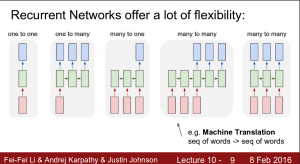A special class of these problems is called a sequence to sequence modelling problem, where the input as well as the output are a sequence. Examples of sequence to sequence problems can be:

1. Machine Translation – An artificial system which translates a sentence from one language to the other.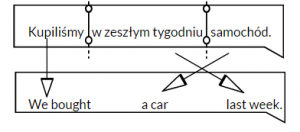2. Video Captioning – Automatically creating the subtitles of a video for each frame, including a description of the sound cues (such as machinery starting up, people laughing in the background, etc.).## A glance of Sequence to Sequence modelling technique

A typical sequence to sequence model has two parts – an encoder and a decoder. Both the parts are practically two different neural network models combined into one giant network.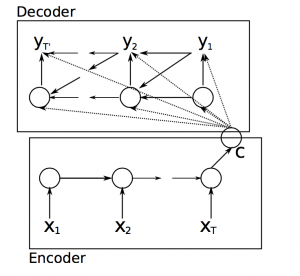Broadly, the task of an encoder network is to understand the input sequence, and create a smaller dimensional representation of it. This representation is then forwarded to a decoder network which generates a sequence of its own that represents the output. Let’s take an example of a conversational agent to understand the concept.Source: https://github.com/farizrahman4u/seq2seq

In the image given above, the input sequence is “How are you”. So when such an input sequence is passed though the encoder-decoder network consisting of LSTM blocks (a type of RNN architecture), the decoder generates words one by one in each time step of the decoder’s iteration. After one whole iteration, the output sequence generated is “I am fine”.

## Improving the performance of models – Beam Search and Attention mechanism

A sequence to sequence modelling network should not be used out of the box. It still needs a bit of tuning to squeeze out the best performance out there to meet expectations. Below are two techniques which have proven to be useful in the past in sequence to sequence modelling applications.

1. Beam Search
2. Attention mechanism

### Beam Search

As we saw before, the decoder network generates the probability of occurrence of a word in the sequence. At each time step, the decoder has to make a decision as to what the next word would be in the sequence.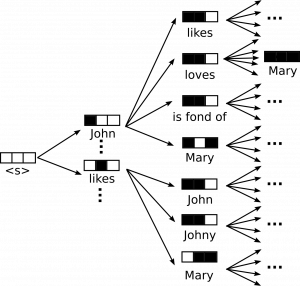One way to make a decision would be to greedily find out the most probable word at each time step.

For example, if the input sequence was “Who does John like?”, there could be many sentences that can be generated by a single decoder network in multiple iterations, making a tree like structure of sentences as shown above. The greedy way would be to pick a word with the greatest probability at each time step.

What if it comes out to be, “likes Mary John”? This does not necessarily give us the sentence with the highest combined probability. For this, you would have to intelligently sort out the appropriate sequence for the sentence.

Beam search takes into account the probability of the next k words in the sequence, and then chooses the proposal with the max combined probability, as seen in the image below: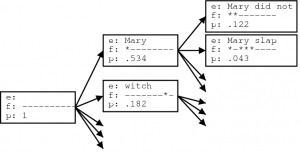### Attention mechanism

When a human tries to understand a picture, he/she focuses on specific portions of the image to get the whole essence of the picture. In the same way, we can train an artificial system to focus on particular elements of the image to get the whole “picture”. This is essentially how attention mechanism works.

Let’s take an example of an image captioning problem, where the system has to generate a suitable caption for an image. In this scenario, to generate the caption, attention mechanism helps the model to grasp individual parts of the image which are most important at that particular instance.To implement attention mechanism, we take input from each time step of the encoder – but give weightage to the timesteps. The weightage depends on the importance of that time step for the decoder to optimally generate the next word in the sequence, as shown in the image below:Keeping these two techniques in mind, you can then build an end-to-end sequence to sequence model that works wonderfully well. In the next section, we will go through a hands on example of the topics we have learnt and apply it on a real life problem using python.

## Hands-on view of Sequence to Sequence modelling

Let’s look at a simple implementation of sequence to sequence modelling in keras. The task is to translate short English sentences into French sentences, character-by-character using a sequence-to-sequence model.

The code for this example can be found on GitHub. The original author of this code is Francois Chollet.

For implementation, we will use a dataset consisting of pairs of English sentences and their French translation, which you can download from here (download the file named `fra-eng.zip`). You also should have keras installed in your system. Here’s a summary of our implementation:

1) Turn the sentences into 3 Numpy arrays, `encoder_input_data``decoder_input_data``decoder_target_data`:

• `encoder_input_data` is a 3D array of shape `(num_pairs, max_english_sentence_length, num_english_characters)` containing a one-hot vectorization of the English sentences
• `decoder_input_data` is a 3D array of shape `(num_pairs, max_french_sentence_length, num_french_characters)` containing a one-hot vectorization of the French sentences
• `decoder_target_data` is the same as `decoder_input_data` but offset by one timestep`decoder_target_data[:, t, :]` will be the same as `decoder_input_data[:, t + 1, :]`

2) Train a basic LSTM-based Seq2Seq model to predict `decoder_target_data` given `encoder_input_data` and `decoder_input_data`.

3) Decode some sentences to check that the model is working (i.e. turn samples from `encoder_input_data` into corresponding samples from `decoder_target_data`).

Now let’s have a look at the python code.

```# import modules
from keras.models import Model
from keras.layers import Input, LSTM, Dense

# Define an input sequence and process it.
encoder_inputs = Input(shape=(None, num_encoder_tokens))
encoder = LSTM(latent_dim, return_state=True)
encoder_outputs, state_h, state_c = encoder(encoder_inputs)
# We discard `encoder_outputs` and only keep the states.
encoder_states = [state_h, state_c]

# Set up the decoder, using `encoder_states` as initial state.
decoder_inputs = Input(shape=(None, num_decoder_tokens))
# We set up our decoder to return full output sequences,
# and to return internal states as well. We don't use the
# return states in the training model, but we will use them in inference.
decoder_lstm = LSTM(latent_dim, return_sequences=True, return_state=True)
decoder_outputs, _, _ = decoder_lstm(decoder_inputs,
initial_state=encoder_states)
decoder_dense = Dense(num_decoder_tokens, activation='softmax')
decoder_outputs = decoder_dense(decoder_outputs)

# Define the model that will turn
# `encoder_input_data` & `decoder_input_data` into `decoder_target_data`
model = Model([encoder_inputs, decoder_inputs], decoder_outputs)

```
```# Run training
model.compile(optimizer='rmsprop', loss='categorical_crossentropy')
model.fit([encoder_input_data, decoder_input_data], decoder_target_data,
batch_size=batch_size,
epochs=epochs,
validation_split=0.2)```

```encoder_model = Model(encoder_inputs, encoder_states)

decoder_state_input_h = Input(shape=(latent_dim,))
decoder_state_input_c = Input(shape=(latent_dim,))
decoder_states_inputs = [decoder_state_input_h, decoder_state_input_c]
decoder_outputs, state_h, state_c = decoder_lstm(
decoder_inputs, initial_state=decoder_states_inputs)
decoder_states = [state_h, state_c]
decoder_outputs = decoder_dense(decoder_outputs)
decoder_model = Model(
[decoder_inputs] + decoder_states_inputs,
[decoder_outputs] + decoder_states)```

```def decode_sequence(input_seq):
# Encode the input as state vectors.
states_value = encoder_model.predict(input_seq)

# Generate empty target sequence of length 1.
target_seq = np.zeros((1, 1, num_decoder_tokens))
# Populate the first character of target sequence with the start character.
target_seq[0, 0, target_token_index['\t']] = 1.

# Sampling loop for a batch of sequences
# (to simplify, here we assume a batch of size 1).
stop_condition = False
decoded_sentence = ''
while not stop_condition:
output_tokens, h, c = decoder_model.predict(
[target_seq] + states_value)

# Sample a token
sampled_token_index = np.argmax(output_tokens[0, -1, :])
sampled_char = reverse_target_char_index[sampled_token_index]
decoded_sentence += sampled_char

# Exit condition: either hit max length
# or find stop character.
if (sampled_char == '\n' or
len(decoded_sentence) > max_decoder_seq_length):
stop_condition = True

# Update the target sequence (of length 1).
target_seq = np.zeros((1, 1, num_decoder_tokens))
target_seq[0, 0, sampled_token_index] = 1.

# Update states
states_value = [h, c]

return decoded_sentence```

Here is a preview of the output which is generated:

```Input sentence: Be nice.
Decoded sentence: Soyez gentil !
-
Input sentence: Drop it!
Decoded sentence: Laissez tomber !
-
Input sentence: Get out!
Decoded sentence: Sortez !```

## End Notes

In this article, we went through a brief overview of sequence to sequence modelling and attention models, two of the most important techniques which are used in state-of-the-art deep learning products focused on natural language and speech processing.

There is a lot of scope of applying these models in practical day-to-day life scenarios. If you do try them out, let us know in the comments below!

### Learn, engage, hack and get hired!###### JalFaizy Shaikh

Faizan is a Data Science enthusiast and a Deep learning rookie. A recent Comp. Sc. undergrad, he aims to utilize his skills to push the boundaries of AI research.

## 5 thoughts on "Essentials of Deep Learning – Sequence to Sequence modelling with Attention (using python)"###### Nandini says:March 15, 2018 at 5:46 pm